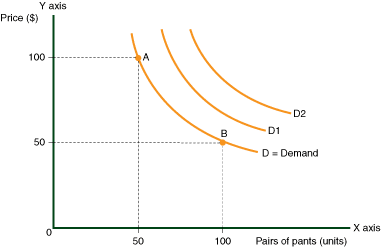# What is a Curve

Understanding What is a Curve:

A curve is simply a type of line drawn upon a graph. For a more detailed explanation, please look at the following diagram.The curve is plotted on two axes:

• The horizontal one is called the X axis. The vertical one is called the Y axis.

• Both axes start at 0. Within the area bordered by these two lines, we can draw either one or several types of curves. Note that even when the actual figures resemble straight lines, economists still refer to them as curves.

Every axis is marked by an ascending scale, and measures a specific feature, for example the price or quantity of a product. Every point on the curve illustrates a relationship between the measures on each axis. If we look at any particular point on the graph, we can see that it relates to both the X and Y axes: There is one measure or mark on the X axis directly below the point on the curve, and another measure or mark on the Y axis directly to the left of the point on the curve. n the following diagram, the line that connects point A to point B displays the data located between these two points.

In every subsequent chapter that includes curves, the relationship between the two axes, and every point in the resulting curve, will be explained in detail as in the following diagram:

• Point A indicates that if the price of trousers in Country A is \$100, then 50 pairs of trousers will be bought.

• Point B shows that if the price of trousers in Country A is \$50, then 100 pairs of trousers will be bought.

The reliability of this data is another question, but the value of graphs is the primary subject under discussion. The purpose of this curve is to illustrate the relationship between price and demand. The lower the price, then the more trousers will be bought. As can be seen, this is a fairly straightforward and simple statement. The usage of curves, however, makes it easier to determine how the price and demand for trousers could be influenced by other events, such as the introduction of new taxes, or imported goods from emerging nations.